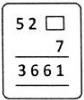Courses

# Test: Multiplication And Division - 1

## 10 Questions MCQ Test Mathematics (Maths) for Class 4 | Test: Multiplication And Division - 1

Description
This mock test of Test: Multiplication And Division - 1 for Class 4 helps you for every Class 4 entrance exam. This contains 10 Multiple Choice Questions for Class 4 Test: Multiplication And Division - 1 (mcq) to study with solutions a complete question bank. The solved questions answers in this Test: Multiplication And Division - 1 quiz give you a good mix of easy questions and tough questions. Class 4 students definitely take this Test: Multiplication And Division - 1 exercise for a better result in the exam. You can find other Test: Multiplication And Division - 1 extra questions, long questions & short questions for Class 4 on EduRev as well by searching above.
QUESTION: 1

### An auditorium had 240 rows of chairs. If there are 170 chairs in each row, how many persons can be seated in the auditorium?

Solution:

No. of chairs in each row = 240 No. of rows of chairs = 170
∴Total no. of chairs =240 × 170=40800
∴ 40800 persons can be seated in the auditorium.

QUESTION: 2

### Identify the missing number. 163 × 38 = (160 + 3) × [ ]

Solution:

163 = 160 + 3 and 38 = 35 + 3 So, 163 × 38=(160 + 3) × (35 + 3)
∴ The missing number is (35 + 3).

QUESTION: 3

### Find the product obtained when the difference between 12030 and 8107 is multiplied by 37.

Solution:

The difference between 12030 and 8107 multiplied by 37 is written as (12030 − 8107) × 37 = 3923 × 37= (3900 + 23) × (40 − 3)

QUESTION: 4

Identify the product of 684 and 43 rounded off to the nearest 100.

Solution:
QUESTION: 5

The total weight of 4 dictionaries and 1079 exercise books is 75 kg. If each exercise book weighs 68 g, what is the weight of each dictionary?

Solution:

total weight = 75 kg = 75000g

since, 1 kg = 1000g

weight of 1 excercise book = 68g

weight of 1079 excercise book = 68 * 1079 = 73372g

so, weight of 4 dictionary = 75000- 73372 = 1628g

therefore, weight of 4 dictionary = 1628/ 4 = 407g

hence the weight of 1 dictionary is 407 grams

QUESTION: 6

The cost of a bicycle is Rs. 797. What is the cost of 8 bicycles?

Solution:
QUESTION: 7

What is the number in [    ] if 8 × 6 = 2 × [ ] × 8?

Solution:

8 × 6 = 2 × 3 × 8 (Since, 6 = 2 × 3)

QUESTION: 8

Sumanth saves Rs. 250 every month. How much money will he be saving in one year?

Solution:

Rs.250 × 12 = Rs. 3000

QUESTION: 9

Which digit should be placed in [  ], so that the following multiplication is correct?Solution:

Since 3 × 7 = 21, and 523 × 7 = 3661, the missing digit is 3.

QUESTION: 10

A school club has 525 members. If each member pays Rs. 11 as the membership fee, how much money is collected?

Solution:

Money collected = Rs. 11 × 525 = Rs. 5775